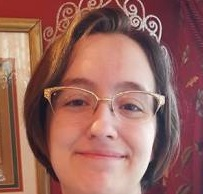# 7.3: Verb Tenses: Passive Voice

•• Contributed by Robing Jeffrey
• Learning Resource Center (LRC) Director at Klamath Community College

## SIMPLE TENSES

### SIMPLE PRESENT

$$\text{General facts or habitual repetitive actions} = \text{am, is, are} + \text{past participle}$$

Example $$\PageIndex{1}$$:

• Lunch is served at noon.
• The locks are checked every night.

### SIMPLE PAST

$$\text{Completed past actions} = \text{was, were} + \text{past participle}$$

Example $$\PageIndex{2}$$:

She was rewarded for her information.

### SIMPLE FUTURE

$$\text{Future promises, predictions, or actions} = \text{will be} + \text{past participle}$$

Example $$\PageIndex{3}$$:

I will be there on Saturday.

## SIMPLE PROGRESSIVE

### PRESENT PROGRESSIVE

$$\text{Future actions (paired with go, leave, move, etc.) or actions that are currently in progress} = \text{am, is, are} + \text{being} + \text{past participle}$$

Example $$\PageIndex{4}$$:

• The votes are being counted by impartial volunteers.
• Joe is being crowned king of the dance.

### PAST PROGRESSIVE

$$\text{Actions that were in progress at a specific time past} = \text{was, were} + \text{being} + \text{past participle}$$

Example $$\PageIndex{5}$$:

They thought they were being careful.

## PERFECT TENSES

### PRESENT PERFECT

$$\text{Actions that happened at an unspecified time in the past or that begin in the past but are still currently occurring} = \text{has, have} + \text{been} + \text{past participle}$$

Example $$\PageIndex{6}$$:

• The boat has been delayed because of the hurricane in the Atlantic.
• Tests have been proctored by teachers for many years.

### PAST PERFECT

$$\text{Actions that began or occurred before something else in the past} = \text{had} + \text{been} + \text{past participle}$$

Example $$\PageIndex{7}$$:

She had been searching for clues for hours before bedtime.

Note

Future progressive, future perfect, & perfect progressive are not used in passive voice.